MORE IN Structural Analysis 1
VTU Civil Engineering (Semester 4)
Structural Analysis 1
June 2015
Total marks: --
Total time: --
INSTRUCTIONS
(1) Assume appropriate data and state your reasons
(2) Marks are given to the right of every question
(3) Draw neat diagrams wherever necessary

1 (a) Distinguish between statically determinate and indeterminate structures with examples.
8 M
1 (b) Free degree of indeterminacy of following structure shown in Fig. 1 (b).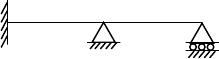(A)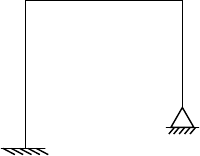(B)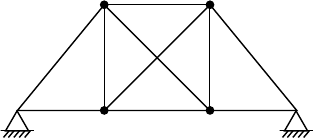(C)

6 M
1 (c) Derive an expression for strain energy stored due to bending.
6 M

2 (a) A cantilever beam of length 4m is loaded as shown in Fig. Q2(a). Calculate the deflection and slope at free end by moment area method. Faking EI is constant.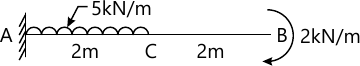10 M
2 (b) For the beam shown in Fig. 2(b). Determine slope at left support and deflection at 100 kN load, using conjugate beam method.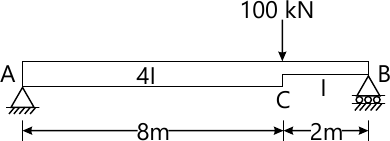10 M

3 (a) Find the vertical deflection at 'C' for the bent shown in Fig. 3(a), by real work method [strain energy]. Take EI is constant.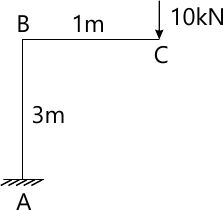10 M
3 (b) Determine the deflection under 60 kN loads in the beam shown in Fig. Q3 (b), by strain energy method.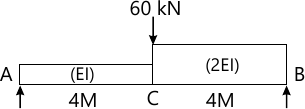10 M

4 (a) Determine the reaction at prop for a propped cantilever beam carrying of UDL of w/unit length throughout span. Take EI is constant using strain energy method.
8 M
4 (b) Analyse the fixed beam strain energy method and draw SFD and BMD. Shown in Fig Q4(b).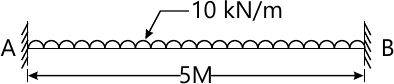12 M

5 (a) A three hinged parabolic arch has a span of 20 mts and rise of 5 mts. It carries a udl of 2 kN/m over the left half of the span and a point load of 12 kN at 5mt from the right end. Find the BM, normal thrust and radial shear at a section 4 mts from left end.
12 M
5 (b) A cable is suspended between two points A and B 100 mts apart and a central dip of 8 mts. It carries udl of 20 kN/m. Find: i) length of the cable ii) maximum and minimum tension in the cable.
8 M

6 (a) Draw SFD and BMD for the propped cantilever beam loaded as shown in Fig. Q6(a). Using consistent deformation method.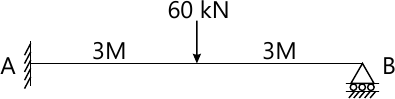10 M
6 (b) Analyse the fixed beam shown in Fig. Q6(b). Draw BMD and SFD by consistent deformation method.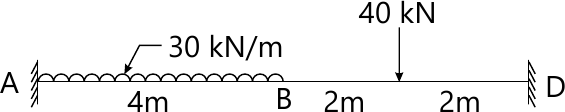10 M

7 Analyse the continuous beam shown in Fig. Q7, by Clapeyron's three moment theorem. Draw SFD and BMD. Take EI is constant.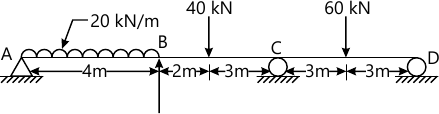20 M

8 Find the horizontal thrust for the wo hinged arch as shown in Fig. Q8. The moment of inertia at any section is Ic sec θ is the slope at section and Ic is MI at the crown. Neglect the effect of rib shortening. Draw BMD.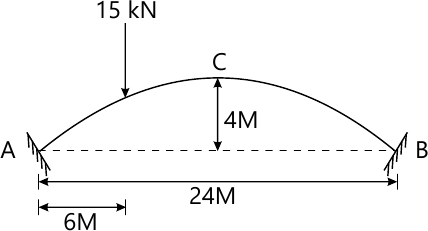20 M

More question papers from Structural Analysis 1